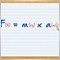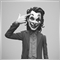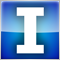# Problems with my moving average crossover EA.33

Hi, I am preparing my first "simple" strategy, but for me it wasn´t that simple... I created this code:

//Global Variables

extern int slow =  9, fast = 3; // for subsequent optimization process **

extern int z= 150, w = 150;

int start()

{

//--------------------------------------------------------Variables declaration.

int pricet = (High+Close+Low)/3;

int ma1 =iMA(NULL, 0, slow, 0,MODE_SMA, PRICE_TYPICAL,0);

int ma2 =iMA(NULL, 0, fast, 0, MODE_SMA, PRICE_TYPICAL,0);

int ma3 =iMA(NULL, 0, slow, 0,MODE_SMA, pricet,0);// I created this variable for simultaneously condition.

int ma4=iMA(NULL, 0, fast, 0, MODE_SMA, pricet,0);

//---------------------------------------------------

if(ma4<ma3 && ma2 == ma1)//simultaneously condition

{

}

//----------------------------------------------------

if(ma4>ma3 && ma2== ma1)// simultaneously condition

{

}

//----------------------------------------------------

return(ticket);

}

When I run it with the strategy tester, it just doesnt execute the OrderSend(). Anyone can help me please :)????16412

theforce:

Hi, I am preparing my first "simple" strategy, but for me it wasn´t that simple... I created this code:

//Global Variables

extern int slow =  9, fast = 3; // for subsequent optimization process **

extern int z= 150, w = 150;

int start()

{

//--------------------------------------------------------Variables declaration.

int pricet = (High+Close+Low)/3;

int ma1 =iMA(NULL, 0, slow, 0,MODE_SMA, PRICE_TYPICAL,0);

int ma2 =iMA(NULL, 0, fast, 0, MODE_SMA, PRICE_TYPICAL,0);

int ma3 =iMA(NULL, 0, slow, 0,MODE_SMA, pricet,0);// I created this variable for simultaneously condition.

int ma4=iMA(NULL, 0, fast, 0, MODE_SMA, pricet,0);

//---------------------------------------------------

if(ma4<ma3 && ma2 == ma1)//simultaneously condition

{

}

//----------------------------------------------------

if(ma4>ma3 && ma2== ma1)// simultaneously condition

{

}

//----------------------------------------------------

return(ticket);

}

When I run it with the strategy tester, it just doesnt execute the OrderSend(). Anyone can help me please :)????

loop in void OnTick()Moderator
20371

Do you have your SL and TP reversed?

Please use the SRC button when posting code, I have done it for you this time.159843

theforce:

Hi, I am preparing my first "simple" strategy, but for me it wasn´t that simple... I created this code:

When I run it with the strategy tester, it just doesnt execute the OrderSend(). Anyone can help me please :)????

Your ma1 and m12 will almost never be equal. It is a bad idea to us a comparison like this
if(ma4<ma3 && ma2 == ma1)
or this :
if(ma4>ma3 && ma2 == ma1)
in the conditions - it will, as you have already noticed, almost never happen. Use some other conditions2021024192

The iMA function has the following structure:

``` iMA (
string        symbol,           // symbol
int           timeframe,         // timeframe
int           ma_period,         // MA averaging period
int           ma_shift,         // MA shift
int           ma_method,         // averaging method
int           applied_price,     // applied price
int           shift             // shift
);```

The parameter applied_price can only take the following values:

PRICE_CLOSE -> 0

PRICE_OPEN -> 1

PRICE_HIGH -> 2

PRICE_LOW -> 3

PRICE_MEDIAN -> 4

PRICE_TYPICAL -> 5

PRICE_WEIGHTED -> 6

In the following code you gave wrong use to the parameter applied_price:

``` int ma3 = iMA ( NULL , 0 , slow, 0 , MODE_SMA , pricet, 0 ); // I created this variable for simultaneously condition.

int ma4= iMA ( NULL , 0 , fast, 0 , MODE_SMA , pricet, 0 );```

You must assign some of the allowed values24192

Try this code

```//Global Variables

extern int slow =  9, fast = 3; // for subsequent optimization process **

extern int z= 150, w = 150;

void OnTick()

{
int ticket;

//--------------------------------------------------------Variables declaration.

int maSlow_0 =iMA(NULL, 0, slow, 0, MODE_SMA, PRICE_TYPICAL,0);

int maFast_0 =iMA(NULL, 0, fast, 0, MODE_SMA, PRICE_TYPICAL,0);

int maSlow_1 =iMA(NULL, 0, slow, 0, MODE_SMA, PRICE_TYPICAL,1);

int maFast_1 =iMA(NULL, 0, fast, 0, MODE_SMA, PRICE_TYPICAL,1);

//---------------------------------------------------

if(maFast_1<maSlow_1 && maFast_0>=maSlow_0)//simultaneously condition

{

}

//----------------------------------------------------

if(maFast_1>maSlow_1 && maFast_0<=maSlow_0)// simultaneously condition

{